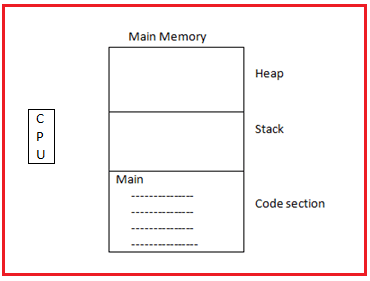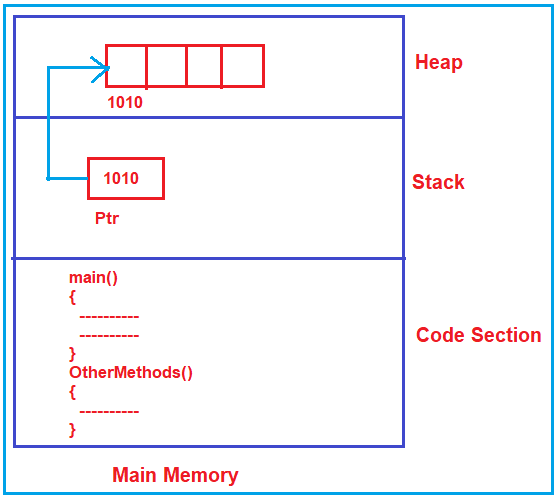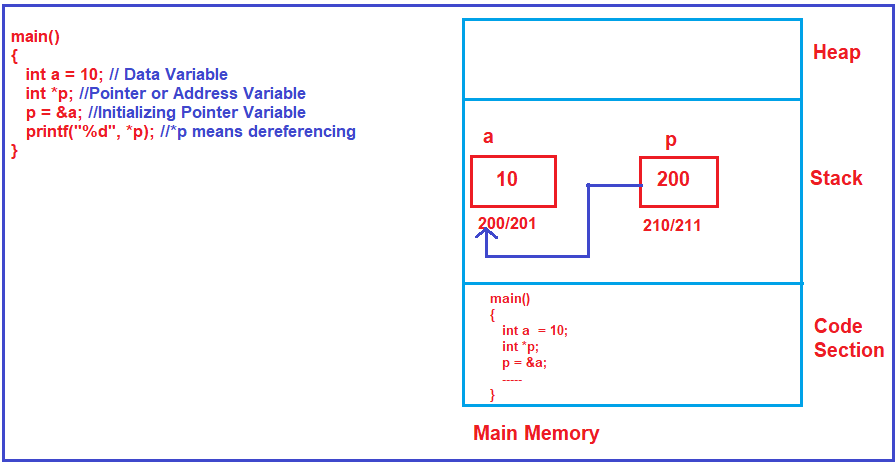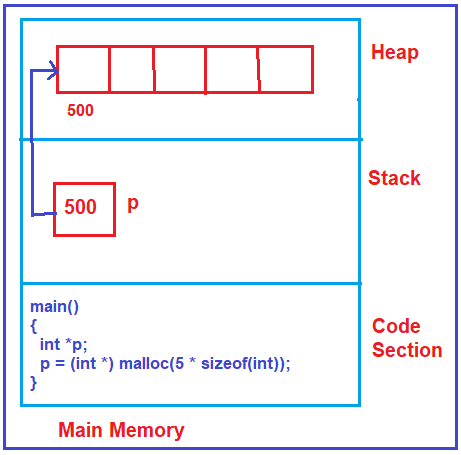# Pointers

## Pointers | Why we should learn Pointers

In this article, I am going to discuss the basics of Pointers which are required to learn data structure and algorithm. Please read our previous article where we discussed the basics of Structures.

##### What are the Pointers?

The pointer is an address variable that is meant for storing the address of data, not the data itself. Normal variables are data variables, but the pointers are address variables, and pointers are used for indirectly accessing the data.

##### Why do we need to access data indirectly?

Let us first understand why do we need pointers and then we will see how to declare and use them. Look at the picture below.As you can see in the above image, there is the main memory and CPU which will execute our programs. As we already discussed in our previous articles, the main memory is divided into three parts i.e. Code Section, Stack, and Heap. Our program code (main function or other functions) reside in the code section.

The program can directly access the Code Section and the Stack section of the Main memory. The program will not directly access the heap. So, the heap memory is external to the program, outside the program and the program doesn’t access directly.

##### How to access the heap area by the program?

To access the heap area by the program we need a pointer. So, one of the reasons for using a pointer is to access the heap area. So, the program should have a pointer with itself, and with that pointer, we can access anything in the heap area such as an array or any other variables. For better understanding, please have a look at the below image.Note: Pointer is useful for accessing the resources that are outside of the program i.e. outside of the code section and heap memory.

##### Other Scenarios where we need Pointers:

Suppose in the hard disk we have some files. Then the program cannot access the hard disk files directly because the hard disk is external or files are external to a program. So, for that, it needs a pointer for accessing and the pointer should be a file type so that it can access that file.

In some scenarios, the program may be accessing a keyboard, the program may be accessing a monitor, or maybe accessing the internet or network connection. All these things are external to a program, all these things can only be accessed with the help of pointers.

So, one major use of pointer is accessing the resources which are outside the program. Pointers are used for accessing heap memory, accessing resources, and parameter passing also.

##### How to declare a pointer, how to initialize, and how to use it?

Let’s take an example. Please have a look at the below image.As you can see in the above image, here we are declaring a data variable ‘a’ and also initialize it with a value 10. Pointer variables are also called address variables in C and C++. Here, *p is a pointer variable. In our example, both a and *p are going to be created in the Stack area of the Main memory. Then we initialize the pointer variable (p = &a) to address the data variable a. So, the pointer now storing the address of a. * is called dereferencing.

##### How to access heap memory using a pointer?

Whenever we declare any variable whether it is a data variable or a pointer variable, it is going to be created inside the Stack frame of the Main memory. So, in the previous example, both the variables are created inside the Stack memory only. Now, the question is how we will get memory in heap?

##### How to get memory in heap?

In the C language, there is a function called malloc(). When you write malloc(), then only you will get the memory in heap. In order to use the malloc() function first you need to include the #include<stdlib.h> header file.

While using the malloc() function we need to specify the size i.e. how many bytes of memory you want in the heap. For example, let say we want memory for 5 integers in the heap. Then we need to specify the size as shown below. Here the sizeof(int) will give the size of an integer in bytes depending on the compiler and operating system you used. If the compiler takes 2 bytes for integer then it will allocate 10 bytes in the heap. If the compiler takes 4 bytes for integer then it will allocate 20 bytes in the heap.

malloc(5 * sizeof(int));

The malloc function returns a void pointer. So, we need to typecast it to an integer pointer as shown below.
int *p;
p = (int *) malloc(5 * sizeof(int));

In C++, you can do the same using a new operator as shown below.
int *p;
p = new int;

For a better understanding of the memory architectures, please have a look at the following image.This is just an introduction to the pointer. As we progress in this course, we will be discussing more and more along with pointer examples.

In the next article, I am going to discuss the References in C++ which is very important to understand data structure and algorithm. Here, in this article, I try to explain the basics of Pointers and I hope you enjoy this article.